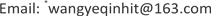1. 引言

2. 嘉兴市居民电动自行车出行特征统计分析2.1. 电动自行车使用者特征

Age and monthly income distribution of electric bicycle use

0~206<200053
20~29712000~500028
30~39115000~800010
40~495>80009
50以上7

2.2. 电动自行车出行特征

2.2.1. 出行频率

2.2.2. 出行目的

Travel characteristics distribution of electric bicycl

3~6次10上学17
7~10次6娱乐25
10次以上8购物16

10~20分钟20接送8
20~30分钟18访友5
30分钟以上10就医0

2.2.3. 出行时长

2.3. 电动自行车出行违章行为分析

2.3.1. 闯红灯行为

Statistic analysis of running a red light behavior of electric bicycle’s trave

2.3.2. 随意变道和抢道

2.3.3. 电动自行车载人情况

2.3.4. 电动自行车超速行为

2.3.5. 电动自行车酒驾问题

3. 电动自行车出行行为建模3.1. 模型描述

Selection and definition of independent variable

V2年龄0~20岁 = 1，20~29岁 = 2，30~39岁 = 3，40~49岁 = 4，50岁以上 = 5
V3职业企业单位人员 = 1，机关事业单位人员 = 2，学生 = 3， 农民 = 4，个体户 = 5，待业人员 = 6，退休人员 = 7，其他 = 8
V4月收入少于2000元 = 1，2000~5000元 = 2，5000~8000元 = 3，8000元以上 = 4

V6出行目的上班 = 1，上学 = 2，娱乐 = 3，购物 = 4，公务 = 5， 接送 = 6，访友 = 7，就医 = 8，其他 = 9
V7出发时刻早高峰(7:00~9:00) = 1，晚高峰(16:00~18:00) = 2，其他 = 3

V9载人行为经常 = 1，偶尔 = 2，一般 = 3，几乎不 = 4，从不 = 5
V10超速行为低速(0 km/h~20 km/h) = 1，低中速(20 km/h~30 km/h) = 2， 中高速(30 km/h~40 km/h) = 3，高速(40 km/h~60 km/h) = 4
V11随意变道或抢道行为经常 = 1，偶尔 = 2，一般 = 3，几乎不 = 4，从不 = 5
V12酒驾行为经常 = 1，偶尔 = 2，一般 = 3，几乎不 = 4，从不 = 5

P n i = exp ( β k X n i ） ∑ j ∈ S i exp ( β k X n j ） (1)

3.2. 模型参数标定

Estimation results of a binary logit model for travel frequency of electric bicycl

_cons−3.150.04−1.070.28
LR chi2(12)42.53
Prob > chi20.00
Pseudo R20.39
Log likelihood−33.84

3.3. 讨论和分析

4. 建议

4.1. 政策制定和执行

2018年新颁布的《电动自行车安全技术规范》 (GB 17761-2018)规定电动自行车出行的速度在0 km/h~25 km/h，而本文研究结果显示嘉兴市有约40%的人存在电动自行车出行超速行为，部分原因由于出行者自身安全意识薄弱，另一部分原因是电动自行车设计时速度较高。电动自行车为人们出行带来便利的同时，其违规行为也给道路交通带来了安全隐患，目前我国电动自行车保有量高达2.5亿辆，法律法规的制定以及执行过程应考虑实际情况，逐步推进。建议对生产、销售超标电动自行车的厂家依法取缔，在交通流量较大的城市地区，应禁止超标电动自行车上路，在交通流量较少范围较大的农村地区应逐步取缔超标电动自行车。

4.2. 交通规划和管理的改善

5. 结论